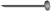# User ForumSubject :IMO    Class : Class 3

Class : Class 1

(D): length of crayon = 3length of key = 2Total length of crayon and key =  3+ 2= 5Subject :IMO    Class : Class 2

Class : Class 1

(C): Amount of water Nitesh drinks in one week = 35 litres

= 5+5+5+5+5+5+5
Amount of water Nitesh drinks each day = 5 litres
Amount of water Nitesh drinks in 10 days = 5+5+5+5+5+5+5+5+5+5 = 50 litres.

Subject :IMO    Class : Class 2

Class : Class 1

(A): Govind : 55 > 52
Neha : 96 < 98
Anusha : 86 < 89
Armaan : 98 < 99
So, Govind scored more in Mathematics than in English.

Subject :IMO    Class : Class 2

Class : Class 1

(A): Number of cards John had = 152
Number of cards Karan had = 129
Number of more cards John had than Karan = 152 - 129 = 23

Subject :IMO    Class : Class 2

Class : Class 1

(B): The clock shows 6 : 40 that is Twenty minutes to 7.

Subject :IMO    Class : Class 2

## Ans 1:

Class : Class 2

Subject :IMO    Class : Class 1

Class : Class 6

## Ans 2:

Class : Class 5

Subject :IMO    Class : Class 1

Class : Class 1

(B): Number of months lie between third and eigth months  = 4

Subject :IMO    Class : Class 2

Class : Class 4

Class : Class 4

## Ans 3:

Class : Class 2
A

Class : Class 1

(A):Subject :IMO    Class : Class 5

## Ans 1:

Class : Class 8
Shaded area = Area of 4 small squares = 144m2Area of 1 small square = 144 Ã· 4 = 36m2Side of small square = = 6mWZ = XY = 3 x 6 = 18mWX = ZY = 4 x 6 = 24mPerimeter of rectangle = 2 x (18 24)= 84cm

Class : Class 1

(A): Shaded area = Area of 4 small squares = 144 sq. m
Area of 1 small square = 144 ÷ 4 = 36 sq. m
Side of small square = 6m
WZ = XY = 3 x 6 = 18m
WX = ZY = 4 x 6 = 24m
Perimeter of rectangle = 2 x (18 + 24)
= 84 m

Class : Class 7